# filter

Filter disturbances through vector autoregression (VAR) model

## Syntax

``Y = filter(Mdl,Z)``
``````[Y,E] = filter(Mdl,Z)``````
``Tbl2 = filter(Mdl,Tbl1,Presample=Presample)``
``[___] = filter(___,Name=Value)``

## Description

example

````Y = filter(Mdl,Z)` returns the numeric array `Y` containing the multivariate response series, which results from filtering the underlying input numeric array `Z` containing the multivariate disturbance series. The series in `Z` are associated with the model innovations process through the fully specified VAR(p) model `Mdl`.```

example

``````[Y,E] = filter(Mdl,Z)``` returns the numeric array containing the multivariate model innovations series `E`.```

example

````Tbl2 = filter(Mdl,Tbl1,Presample=Presample)` returns the table or timetable `Tbl2` containing the multivariate response series, which results from filtering the underlying multivariate disturbance series in the input table or timetable `Tbl1`. `filter` initializes the response series using the required table or timetable of presample data in `Presample`. Variables in `Tbl1` are associated with the model innovations process through `Mdl`. (since R2022b)`filter` selects the variables in `Mdl.SeriesNames` or all variables in `Tbl1`. To select different disturbance variables in `Tbl1` to filter through the model, use the `DisturbanceVariables` name-value argument. `filter` selects the same variables for `Presample` by default, but you can select different variables by using the `PresampleResponseVariables` name-value argument.```

example

````[___] = filter(___,Name=Value)` specifies options using one or more name-value arguments in addition to any of the input argument combinations in previous syntaxes. `filter` returns the output argument combination for the corresponding input arguments. For example, `filter(Mdl,Z,Y0=PS,X=Exo)` filters the numeric array of disturbances `Z` through the VAR(p) model `Mdl`, and specifies the numeric array of presample response data `PS` and the numeric matrix of exogenous predictor data `Exo` for the model regression component.```

## Examples

collapse all

Fit a VAR(4) model to the consumer price index (CPI) and unemployment rate data. Then, simulate responses by filtering a random series of Gaussian distributed disturbances through the estimated model. Supply the disturbances as a numeric matrix.

Load the `Data_USEconModel` data set.

`load Data_USEconModel`

Plot the two series on separate plots.

```figure; plot(DataTimeTable.Time,DataTimeTable.CPIAUCSL); title("Consumer Price Index") ylabel("Index") xlabel("Date")``````figure; plot(DataTimeTable.Time,DataTimeTable.UNRATE); title("Unemployment Rate") ylabel("Percent") xlabel("Date")```Stabilize the CPI by converting it to a series of growth rates, include a missing value at the beginning of the series to maintain time consistency among the series. Create a new data set containing the transformed variables, and remove all rows containing at least one missing observation.

```RCPI = [NaN; price2ret(DataTimeTable.CPIAUCSL)]; UNRATE = DataTimeTable.UNRATE; DTT = timetable(RCPI,UNRATE,RowTimes=DataTimeTable.Time); DTT = rmmissing(DTT);```

Create a default VAR(4) model by using the shorthand syntax.

`Mdl = varm(2,4);`

Estimate the model using the entire data set.

`EstMdl = estimate(Mdl,DTT.Variables);`

`EstMdl` is a fully specified, estimated `varm` model object.

Generate a `numobs`-by-2 series of random Gaussian distributed values, where `numobs` is the number of observations in the data.

```numobs = size(DTT,1); rng(1) % For reproducibility Z = mvnrnd(zeros(Mdl.NumSeries,1),eye(Mdl.NumSeries),numobs);```

To simulate responses, filter the disturbances through the estimated model.

`Y = filter(EstMdl,Z);`

`Y` is a 245-by-2 matrix of simulated responses. The first and second columns contain the simulated CPI growth rate and unemployment rate, respectively.

Plot the simulated and true responses.

```figure plot(DTT.Time,Y(:,1)) hold on plot(DTT.Time,DTT.RCPI) ylabel("Growth Rate") xlabel("Date") title("CPI Growth Rate") legend("Simulation","True")```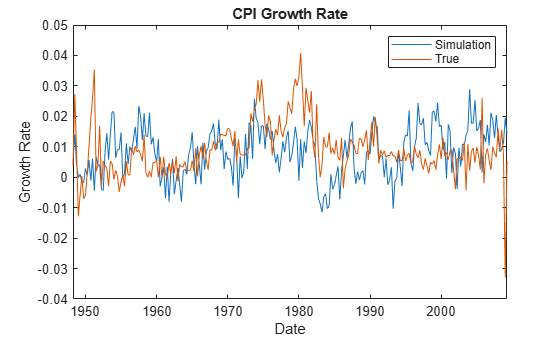```figure plot(DTT.Time,Y(:,2)) hold on plot(DTT.Time,DTT.UNRATE) ylabel("Percent") xlabel("Date") title("Unemployment Rate") legend("Simulation","True") hold off```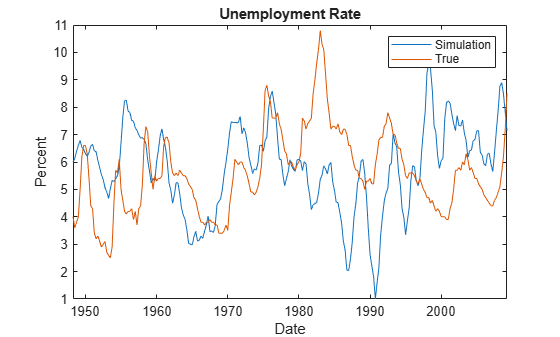Estimate a VAR(4) model of the consumer price index (CPI), the unemployment rate, and the gross domestic product (GDP). Include a linear regression component containing the current quarter and the last four quarters of government consumption expenditures and investment (GCE). Pass multiple multivariate Gaussian disturbance paths through the estimated model.

Load the `Data_USEconModel` data set. Compute the real GDP.

```load Data_USEconModel DataTimeTable.RGDP = DataTimeTable.GDP./DataTimeTable.GDPDEF*100;```

Plot all variables on separate plots.

```figure tiledlayout(2,2) nexttile plot(DataTimeTable.Time,DataTimeTable.CPIAUCSL); ylabel('Index') title('Consumer Price Index') nexttile plot(DataTimeTable.Time,DataTimeTable.UNRATE); ylabel('Percent') title('Unemployment Rate') nexttile plot(DataTimeTable.Time,DataTimeTable.RGDP); ylabel('Output') title('Real Gross Domestic Product') nexttile plot(DataTimeTable.Time,DataTimeTable.GCE); ylabel('Billions of \$') title('Government Expenditures')```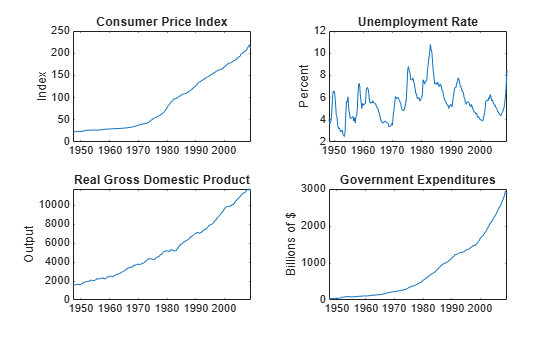Stabilize the CPI, GDP, and GCE by converting each to a series of growth rates. Synchronize the unemployment rate series with the others by removing its first observation.

```inputVariables = {'CPIAUCSL' 'RGDP' 'GCE'}; Data = varfun(@price2ret,DataTimeTable,'InputVariables',inputVariables); Data.Properties.VariableNames = inputVariables; Data.UNRATE = DataTimeTable.UNRATE(2:end);```

Expand the GCE rate series to a matrix that includes its current value and up through four lagged values. Remove the `GCE` variable from `Data`.

```rgcelag4 = lagmatrix(Data.GCE,0:4); Data.GCE = [];```

Create a default VAR(4) model by using the shorthand syntax.

```Mdl = varm(3,4); Mdl.SeriesNames = {'rcpi' 'unrate' 'rgdpg'};```

Estimate the model using the entire sample. Specify the GCE matrix as data for the regression component.

`EstMdl = estimate(Mdl,Data.Variables,'X',rgcelag4);`

Generate 1000 paths of `numobs` observations from a 3-D Gaussian distribution. `numobs` is the number of observations in the data without any missing values.

```numpaths = 1000; numseries = Mdl.NumSeries; idx = all(~ismissing([Data array2table(rgcelag4)]),2); numobs = sum(idx); rng(1); Z = mvnrnd(zeros(Mdl.NumSeries,1),eye(Mdl.NumSeries),numobs*numpaths); Z = reshape(Z,[numobs,3,numpaths]);```

Filter the disturbances through the estimated model. Supply the predictor data. Return the innovations (scaled disturbances).

`[Y,E] = filter(EstMdl,Z,'X',rgcelag4);`

`Y` and `E` are 244-by-3-by-1000 matrices of filtered responses and scaled disturbances, respectively. The columns correspond to the CPI growth rate, unemployment rate, and GDP growth rate, respectively. `filter` applies the same predictor data to all paths.

For each time point, compute the mean vector of the filtered responses among all paths.

`MeanFilt = mean(Y,3);`

`MeanFilt` is a 244-by-3 matrix containing the average of the responses at each time point.

Plot the filtered responses, their averages, and the data.

```Data = Data(idx,:); figure tiledlayout(2,2) for j = 1:Mdl.NumSeries nexttile plot(Data.Time,squeeze(Y(:,j,:)),'Color',[0.8,0.8,0.8]) title(Mdl.SeriesNames{j}); hold on h1 = plot(Data.Time,Data{:,j}); h2 = plot(Data.Time,MeanFilt(:,j)); hold off end hl = legend([h1 h2],'Data','Mean'); hl.Location = 'none'; hl.Position = [0.6 0.25 hl.Position(3:4)];```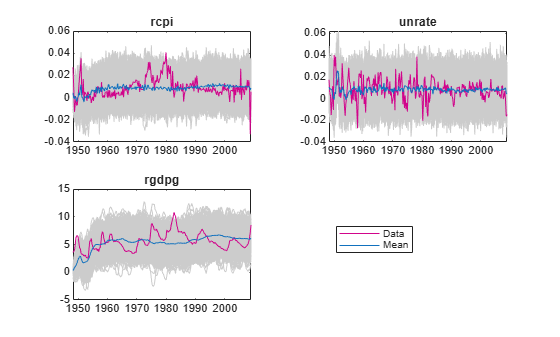Since R2022b

Fit a VAR(4) model to the consumer price index (CPI) and unemployment rate data. Then, simulate responses by filtering multiple random paths of Gaussian distributed disturbances through the estimated model. Supply the disturbances in a timetable. This example is based on Filter Numeric Matrix of Disturbances Through VAR(4) Model.

Load the `Data_USEconModel` data set.

`load Data_USEconModel`

Preprocess the data. Regularize the time base.

```RCPI = [NaN; price2ret(DataTimeTable.CPIAUCSL)]; UNRATE = DataTimeTable.UNRATE; DTT = timetable(RCPI,UNRATE,RowTimes=DataTimeTable.Time); DTT = rmmissing(DTT); dt = DTT.Time; dt = dateshift(dt,"start","quarter"); DTT.Time = dt;```

Fit Model to Data

Estimate the model using the entire data set.

```Mdl = varm(2,4); Mdl.SeriesNames = DTT.Properties.VariableNames; EstMdl = estimate(Mdl,DTT);```

Simulate Paths of Disturbances

Generate a `numobs`-by-`numseries`-by-`numpaths` array of independent random Gaussian distributed values, where `numobs` is the number of observations in the data, `numseries` the number of response series 2, and `numpaths` is 100.

```rng(1) % For reproducibility numobs = height(DTT); numseries = EstMdl.NumSeries; numpaths = 100; Z = mvnrnd(zeros(numseries,1),eye(numseries),numobs*numpaths); Z = reshape(Z,numobs,numseries,numpaths); DTT.ZRCPI = squeeze(Z(:,1,:)); DTT.ZUNRATE = squeeze(Z(:,2,:)); head(DTT)```
``` Time RCPI UNRATE ZRCPI ZUNRATE _____ __________ ______ ____________ ____________ Q1-48 0.0038371 4 1x100 double 1x100 double Q2-48 0.027284 3.6 1x100 double 1x100 double Q3-48 0.0086581 3.8 1x100 double 1x100 double Q4-48 -0.012807 4 1x100 double 1x100 double Q1-49 -0.0058382 5 1x100 double 1x100 double Q2-49 0.00041815 6.2 1x100 double 1x100 double Q3-49 -0.0071324 6.6 1x100 double 1x100 double Q4-49 -0.0059122 6.6 1x100 double 1x100 double ```

Filter Disturbances Through Model

When you filter disturbances by using a timetable, `filter` requires a presample. Split the timetable into presample and in-sample data sets. The presample data is the initial `EstMdl.P` observations, and the in-sample data set contains the remaining observations.

```Presample = DTT(1:EstMdl.P,:); InSample = DTT((EstMdl.P + 1):end,:);```

Simulate response paths by filtering the in-sample disturbances through the estimated model. Specify the variable names of the disturbance series, the presample data, and the response variable names in the presample.

```Tbl2 = filter(EstMdl,InSample,DisturbanceVariables=["ZRCPI" "ZUNRATE"], ... Presample=Presample,PresampleResponseVariables=EstMdl.SeriesNames); size(Tbl2)```
```ans = 1×2 241 8 ```
`head(Tbl2)`
``` Time RCPI UNRATE ZRCPI ZUNRATE RCPI_Responses UNRATE_Responses RCPI_Innovations UNRATE_Innovations _____ __________ ______ ____________ ____________ ______________ ________________ ________________ __________________ Q1-49 -0.0058382 5 1x100 double 1x100 double 1x100 double 1x100 double 1x100 double 1x100 double Q2-49 0.00041815 6.2 1x100 double 1x100 double 1x100 double 1x100 double 1x100 double 1x100 double Q3-49 -0.0071324 6.6 1x100 double 1x100 double 1x100 double 1x100 double 1x100 double 1x100 double Q4-49 -0.0059122 6.6 1x100 double 1x100 double 1x100 double 1x100 double 1x100 double 1x100 double Q1-50 0.0012698 6.3 1x100 double 1x100 double 1x100 double 1x100 double 1x100 double 1x100 double Q2-50 0.010101 5.4 1x100 double 1x100 double 1x100 double 1x100 double 1x100 double 1x100 double Q3-50 0.01908 4.4 1x100 double 1x100 double 1x100 double 1x100 double 1x100 double 1x100 double Q4-50 0.025954 4.3 1x100 double 1x100 double 1x100 double 1x100 double 1x100 double 1x100 double ```

`Tbl2` is a 241-by-2 matrix of in-sample data, paths of simulated disturbances, paths of filtered responses (variables names appended with `_Responses`), and paths of innovations (variables with name appended with `_Innovations`).

Plot the paths of simulated responses with the true responses.

```figure p1 = plot(Tbl2.Time,Tbl2.RCPI_Responses,Color=[0.5 0.5 0.5]); hold on p2 = plot(Tbl2.Time,Tbl2.RCPI,LineWidth=2); ylabel("Growth Rate") xlabel("Date") title("CPI Growth Rate") legend([p1(1) p2],["Simulated" "Observed"]) hold off```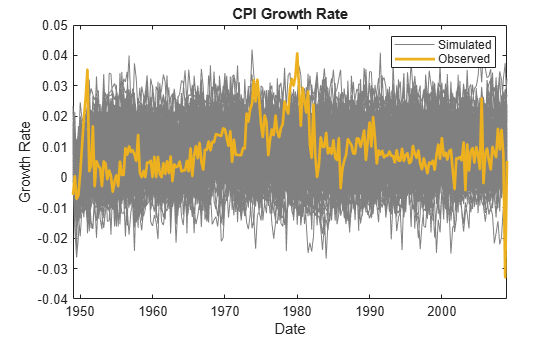```figure p1 = plot(Tbl2.Time,Tbl2.UNRATE_Responses,Color=[0.5 0.5 0.5]); hold on p2 = plot(Tbl2.Time,Tbl2.UNRATE,LineWidth=2); ylabel("Percent") xlabel("Date") title("Unemployment Rate") legend([p1(1) p2],["Simulated" "Observed"]) hold off```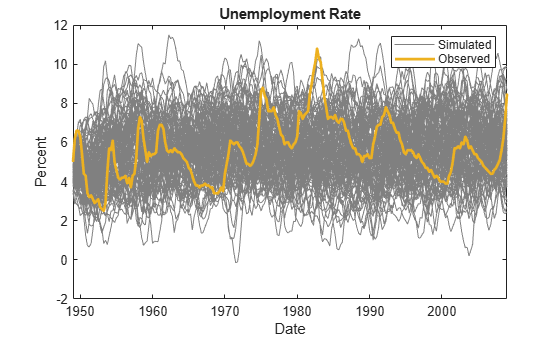## Input Arguments

collapse all

VAR model, specified as a `varm` model object created by `varm` or `estimate`. `Mdl` must be fully specified.

Underlying multivariate disturbance series zt associated with the model innovations process εt, specified as a `numobs`-by-`numseries` numeric matrix or a `numobs`-by-`numseries`-by-`numpaths` numeric array.

`numobs` is the sample size. `numseries` is the number of disturbance series (`Mdl.NumSeries`). `numpaths` is the number of disturbance paths.

Rows correspond to sampling times, and the last row contains the latest set of disturbances.

Columns correspond to individual disturbance series for response variables.

Pages correspond to separate, independent paths. For a numeric matrix, `Z` is a single `numseries`-dimensional path of disturbance series. For a 3-D array, each page of `Z` represents a separate `numseries`-dimensional path. Among all pages, disturbances in corresponding rows occur at the same time.

The `Scale` name-value argument specifies whether to scale the disturbances before `filter` filters them through `Mdl`. For more details, see `Scale`.

Data Types: `double`

Since R2022b

Time series data containing observed disturbance variables zt, associated with the model innovations process εt, or predictor variables xt, specified as a table or timetable with `numvars` variables and `numobs` rows. You can optionally select `numseries` disturbance variables or `numpreds` predictor variables by using the `DisturbanceVariables` or `PredictorVariables` name-value arguments, respectively.

Each selected disturbance variable is a `numobs`-by-`numpaths` numeric matrix, and each predictor variable is a numeric vector. Each row is an observation, and measurements in each row occur simultaneously.

Each path (column) of a disturbance variable is independent of all others, but path `j` of all presample and in-sample variables correspond, for `j` = 1,…,`numpaths`. Each selected predictor variable contains one path, which `filter` applies to all paths.

If `Tbl1` is a timetable, it must represent a sample with a regular datetime time step (see `isregular`), and the datetime vector `Tbl1.Time` must be ascending or descending.

If `Tbl1` is a table, the last row contains the latest observation.

The `Scale` name-value argument specifies whether to scale the disturbances before `filter` filters them through `Mdl`. For more details, see `Scale`.

Since R2022b

Presample data that provides initial values for the model `Mdl`, specified as a table or timetable, the same type as `Tbl1`, with `numprevars` variables and `numpreobs` rows. `Presample` is required when you supply a table or timetable of data `Tbl1`.

Each row is a presample observation, and measurements in each row, among all paths, occur simultaneously. `numpreobs` must be at least `Mdl.P`. If you supply more rows than necessary, `filter` uses the latest `Mdl.P` observations only.

Each variable is a `numpreobs`-by-`numprepaths` numeric matrix. Variables correspond to the response series associated with the respective disturbance in `Tbl1`. To control presample variable selection, see the optional `PresampleResponseVariables` name-value argument.

For each variable, columns are separate, independent paths.

• If variables are vectors, `filter` applies them to each path in `Tbl1` to produce the filtered responses in `Tbl2`. Therefore, all paths of filtered responses derive from common initial conditions.

• Otherwise, for each variable `Vark` and each path `j`, `filter` applies `Presample.Vark(:,j)` to produce `Tbl2.Vark(:,j)`. Variables must have at least `numpaths` columns, and `filter` uses only the first `numpaths` columns.

If `Presample` is a timetable, all the following conditions must be true:

• `Presample` must represent a sample with a regular datetime time step (see `isregular`).

• The inputs `Tbl1` and `Presample` must be consistent in time such that `Presample` immediately precedes `Tbl1` with respect to the sampling frequency and order.

• The datetime vector of sample timestamps `Presample.Time` must be ascending or descending.

If `Presample` is a table, the last row contains the latest presample observation.

### Name-Value Arguments

Specify optional pairs of arguments as `Name1=Value1,...,NameN=ValueN`, where `Name` is the argument name and `Value` is the corresponding value. Name-value arguments must appear after other arguments, but the order of the pairs does not matter.

Before R2021a, use commas to separate each name and value, and enclose `Name` in quotes.

Example: `filter(Mdl,Z,Y0=PS,X=Exo)` filters the numeric array of disturbances `Z` through the VAR(p) model `Mdl`, and specifies the numeric array of presample response data `PS` and the numeric matrix of exogenous predictor data `Exo` for the model regression component.

Since R2022b

Variables to select from `Tbl1` to treat as disturbance variables zt, specified as one of the following data types:

• String vector or cell vector of character vectors containing `numseries` variable names in `Tbl1.Properties.VariableNames`

• A length `numseries` vector of unique indices (integers) of variables to select from `Tbl1.Properties.VariableNames`

• A length `numvars` logical vector, where ```DisturbanceVariables(j) = true``` selects variable `j` from `Tbl1.Properties.VariableNames`, and `sum(DisturbanceVariables)` is `numseries`

The selected variables must be numeric vectors (single path) or matrices (columns represent multiple independent paths) of the same width, and cannot contain missing values (`NaN`).

If the number of variables in `Tbl1` matches `Mdl.NumSeries`, the default specifies all variables in `Tbl1`. If the number of variables in `Tbl1` exceeds `Mdl.NumSeries`, the default matches variables in `Tbl1` to names in `Mdl.SeriesNames`.

Example: `DisturbanceVariables=["GDP" "CPI"]`

Example: `DisturbanceVariables=[true false true false]` or `DisturbanceVariable=[1 3]` selects the first and third table variables as the disturbance variables.

Data Types: `double` | `logical` | `char` | `cell` | `string`

Presample responses that provide initial values for the model `Mdl`, specified as a `numpreobs`-by-`numseries` numeric matrix or a `numpreobs`-by-`numseries`-by-`numprepaths` numeric array. Use `Y0` only when you supply a numeric array of disturbance data `Z`.

`numpreobs` is the number of presample observations. `numprepaths` is the number of presample response paths.

Each row is a presample observation, and measurements in each row, among all pages, occur simultaneously. The last row contains the latest presample observation. `Y0` must have at least `Mdl.P` rows. If you supply more rows than necessary, `filter` uses the latest `Mdl.P` observations only.

Each column corresponds to the response series associated with the respective disturbance in `Z`.

Pages correspond to separate, independent paths.

• If `Y0` is a matrix, `filter` applies it to each path (page) to produce the filtered responses `Y`. Therefore, all paths in `Y` derive from common initial conditions.

• Otherwise, `filter` applies `Y0(:,:,j)` to produce `Y(:,:,j)`. `Y0` must have at least `numpaths` pages, and `filter` uses only the first `numpaths` pages.

By default, `filter` sets any necessary presample observations.

• For stationary VAR processes without regression components, `filter` uses the unconditional mean $\mu ={\Phi }^{-1}\left(L\right)c.$

• For nonstationary processes or models containing a regression component, `filter` sets presample observations to an array composed of zeros.

Data Types: `double`

Since R2022b

Variables to select from `Presample` to use for presample data, specified as one of the following data types:

• String vector or cell vector of character vectors containing `numseries` variable names in `Presample.Properties.VariableNames`

• A length `numseries` vector of unique indices (integers) of variables to select from `Presample.Properties.VariableNames`

• A length `numvars` logical vector, where ```PresampleResponseVariables(j) = true ``` selects variable `j` from `Presample.Properties.VariableNames`, and `sum(PresampleResponseVariables)` is `numseries`

The selected variables must be numeric vectors (single path) or matrices (columns represent multiple independent paths) of the same width, and cannot contain missing values (`NaN`).

`PresampleResponseNames` does not need to contain the same names as in `Tbl1`; `filter` uses the data in selected variable `PresampleResponseVariables(j)` as a presample for the response variable corresponding to `DisturbanceVariables(j)`.

The default specifies the same response variables as those selected from `Tbl1` (see `DisturbanceVariables`).

Example: `PresampleResponseVariables=["GDP" "CPI"]`

Example: `PresampleResponseVariables=[true false true false]` or `PresampleResponseVariable=[1 3]` selects the first and third table variables for presample data.

Data Types: `double` | `logical` | `char` | `cell` | `string`

Predictor data xt for the regression component in the model, specified as a numeric matrix containing `numpreds` columns. Use `X` only when you supply a numeric array of disturbance data `Z`.

`numpreds` is the number of predictor variables (`size(Mdl.Beta,2)`).

Each row corresponds to an observation, and measurements in each row occur simultaneously. The last row contains the latest observation. `X` must have at least as many observations as `Z`. If you supply more rows than necessary, `filter` uses only the latest observations. `filter` does not use the regression component in the presample period.

Each column is an individual predictor variable. All predictor variables are present in the regression component of each response equation.

`filter` applies `X` to each path (page) in `Z`; that is, `X` represents one path of observed predictors.

By default, `filter` excludes the regression component, regardless of its presence in `Mdl`.

Data Types: `double`

Since R2022b

Variables to select from `Tbl1` to treat as exogenous predictor variables xt, specified as one of the following data types:

• String vector or cell vector of character vectors containing `numpreds` variable names in `Tbl1.Properties.VariableNames`

• A length `numpreds` vector of unique indices (integers) of variables to select from `Tbl1.Properties.VariableNames`

• A length `numvars` logical vector, where `PredictorVariables(j) = true ` selects variable `j` from `Tbl1.Properties.VariableNames`, and `sum(PredictorVariables)` is `numpreds`

The selected variables must be numeric vectors and cannot contain missing values (`NaN`).

By default, `filter` excludes the regression component, regardless of its presence in `Mdl`.

Example: `PredictorVariables=["M1SL" "TB3MS" "UNRATE"]`

Example: `PredictorVariables=[true false true false]` or `PredictorVariable=[1 3]` selects the first and third table variables to supply the predictor data.

Data Types: `double` | `logical` | `char` | `cell` | `string`

Flag indicating whether to scale disturbances by the lower triangular Cholesky factor of the model covariance matrix, specified as a value in this table. In the table:

ValueDescription
`true``E(:,:,j)` = `L*Z(:,:,j)`, where `L` = `chol(Mdl.Covariance,"lower")`
`false`No scale, `E(:,:,j)` = `Z(:,:,j)`

For each page `j` = 1,...,`numpaths`, `filter` filters the `numobs`-by-`numseries` matrix of innovations `E(:,:,j)` through the VAR(p) model `Mdl` using the specified scale.

Example: `Scale=false`

Data Types: `logical`

Note

• `NaN` values in `Z`, `Y0`, and `X` indicate missing values. `filter` removes missing values from the data by list-wise deletion.

1. If `Z` is a 3-D array, then `filter` horizontally concatenates the pages of `Z` to form a `numobs`-by-`numpaths*numseries` matrix.

2. If a regression component is present, then `filter` horizontally concatenates `X` to `Z` to form a `numobs`-by-```(numpaths*numseries + numpreds)``` matrix. `filter` assumes that the last rows of each series occur at the same time.

3. `filter` removes any row that contains at least one `NaN` from the concatenated data.

4. `filter` applies steps 1 and 3 to the presample paths in `Y0`.

This process ensures that the filtered responses and innovations of each path are the same size and are based on the same observation times. In the case of missing observations, the results obtained from multiple paths of `Z` can differ from the results obtained from each path individually.

This data reduction reduces the effective sample size.

• `filter` issues an error when any table or timetable input contains missing values.

## Output Arguments

collapse all

Filtered multivariate response series yt, returned as a `numobs`-by-`numseries` numeric matrix or a `numobs`-by-`numseries`-by-`numpaths` numeric array. `Y` represents the continuation of the presample responses in `Y0`.

`filter` returns `Y` only when you supply the input `Z`.

Multivariate model innovations series εt, returned as a `numobs`-by-`numseries` numeric matrix or a `numobs`-by-`numseries`-by-`numpaths` numeric array. For details on the value of `E`, see `Scale`.

`filter` returns `E` only when you supply the input `Z`.

Since R2022b

Multivariate filtered response yt and innovation series εt, returned as a table or timetable, the same data type as `Tbl1`. `filter` returns `Tbl2` only when you supply the input `Tbl1`.

`Tbl2` contains the following variables:

• The filtered response variables yt. Each filtered response variable is a `numobs`-by-`numpaths` numeric matrix, with rows representing observations and columns representing independent paths, each corresponding to the input observations and paths in `Tbl1`. `filter` names the filtered response for disturbance variable `DisturbanceJ` in `Tbl1` `DisturbanceJ_Responses`. For example, if one of the selected disturbance variables in `Tbl1` to filter is `GDP`, `Tbl2` contains a variable for the corresponding filtered responses with the name `GDP_Responses`.

• The innovation variables εt. Each innovation variable is a `numobs`-by-`numpaths` numeric matrix, with rows representing observations and columns representing independent paths, each corresponding to the input observations and paths in `Tbl1`. `filter` names the innovation variable for disturbance variable `DisturbanceJ` in `Tbl1` `DisturbanceJ_Innovations`. For example, if one of the selected disturbance variables in `Tbl1` to filter is `GDP`, `Tbl2` contains a variable for the corresponding innovations with the name `GDP_Innovations`.

• All variables `Tbl1`.

If `Tbl1` is a timetable, `Tbl1` and `Tbl2` have the same row order, either ascending or descending.

## Algorithms

• `filter` computes filtered responses `Y` and innovations `E` using this process for each page `j` in `Z`.

1. If `Scale` is `true`, then `E(:,:,j)` = `L*Z(:,:,j)`, where `L` = `chol(Mdl.Covariance,'lower')`. Otherwise, `E(:,:,j)` = `Z(:,:,j)`. Set et = `E(:,:,j)`.

2. `Y(:,:,j)` is yt in this system of equations.

`${y}_{t}={\stackrel{^}{\Phi }}^{-1}\left(L\right)\left(\stackrel{^}{c}+\stackrel{^}{\delta }t+{e}_{t}\right).$`

For variable definitions, see More About.

• `filter` generalizes `simulate`. Both functions filter a disturbance series through a model to produce responses and innovations. However, whereas `simulate` generates a series of mean-zero, unit-variance, independent Gaussian disturbances `Z` to form innovations `E` = `L*Z`, `filter` enables you to supply disturbances from any distribution.

• `filter` uses this process to determine the time origin t0 of models that include linear time trends:

• If you specify `Z` and you do not specify a presample by using the `Y0` name-value argument, t0 = 0.

• Otherwise, if you specify `Tbl1` or you supply a presample, `filter` sets t0 to `size(Y0,1)``Mdl.P`, where `Y0` contains presample data, the value of `Y0` or `Presample`. Therefore, the times in the trend component are t = t0 + 1, t0 + 2,..., t0 + `numobs`, where `numobs` is the effective sample size (`size(Z,1)`, after `filter` removes missing values, or `height(Tbl1)`). This convention is consistent with the default behavior of model estimation, in which `estimate` removes the first `Mdl.P` responses, reducing the effective sample size. Although `filter` explicitly uses the first `Mdl.P` presample responses in `Y0` to initialize the model, the total number of observations in `Y0` and the input data (`Z`, excluding missing values, or `Tbl1`) determines t0.

 Hamilton, James D. Time Series Analysis. Princeton, NJ: Princeton University Press, 1994.

 Johansen, S. Likelihood-Based Inference in Cointegrated Vector Autoregressive Models. Oxford: Oxford University Press, 1995.

 Juselius, K. The Cointegrated VAR Model. Oxford: Oxford University Press, 2006.

 Lütkepohl, H. New Introduction to Multiple Time Series Analysis. Berlin: Springer, 2005.